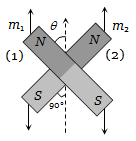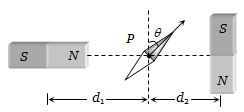If the angular momentum of an electron is $\stackrel{\to }{J}$ then the magnitude of the magnetic moment will be

1. $\frac{eJ}{m}$                            2. $\frac{eJ}{2m}$

3. ej.2m                         4. $\frac{2m}{eJ}$

High Yielding Test Series + Question Bank - NEET 2020

Difficulty Level:

The basic magnetization curve for a ferromagnetic material is shown in the figure. Then, the value of relative permeability is highest for the point :1. P

2. Q

3. R

4. S

Concept Questions :-

Magnetization and magnetic intensity
High Yielding Test Series + Question Bank - NEET 2020

Difficulty Level:

A superconductor exhibits perfect :
(a) Ferrimagnetism               (b) Ferromagnetism
(c) Paramagnetism               (d) Diamagnetism

Concept Questions :-

Magnetic materials
High Yielding Test Series + Question Bank - NEET 2020

Difficulty Level:

Among the following properties describing diamagnetism identify  the property that is wrongly stated

1. Diamagnetic material do not have permanent magnetic moment

2. Diamagnetism is explained in terms of electromagnetic induction

3. Diamagnetic materials have a small positive susceptibility

4. The magnetic moment of individual electrons neutralize each other

Concept Questions :-

Magnetic materials
High Yielding Test Series + Question Bank - NEET 2020

Difficulty Level:

If a magnet is suspended at an angle 30o to the magnetic meridian, it makes an angle of 45o with the horizontal. The real dip is

(a)${\mathrm{tan}}^{-1}\left(\frac{\sqrt{3}}{2}\right)$

(b)${\mathrm{tan}}^{-1}\left(\sqrt{3}\right)$

(c)${\mathrm{tan}}^{-1}\left(\sqrt{\frac{3}{2}}\right)$

(d)${\mathrm{tan}}^{-1}\left(\frac{2}{\sqrt{3}}\right)$

Concept Questions :-

Earth's magnetism
High Yielding Test Series + Question Bank - NEET 2020

Difficulty Level:

The true value of angle of dip at a place is 60o, the apparent dip in a plane inclined at an angle of 30o with magnetic meridian is

(a)${\mathrm{tan}}^{-1}\left(\frac{1}{2}\right)$

(b)${\mathrm{tan}}^{-1}\left(2\right)$

(c)${\mathrm{tan}}^{-1}\left(\frac{2}{3}\right)$

(d)None of these

Concept Questions :-

Earth's magnetism
High Yielding Test Series + Question Bank - NEET 2020

Difficulty Level:

A vibration magnetometer consists of two identical bar magnets placed one over the other such that they are perpendicular and bisect each other. The time period of oscillation in a horizontal magnetic field is ${2}^{5}{4}}$ seconds. One of the magnets is removed and if the other magnet oscillates in the same field, then the time period in seconds is :

(a) ${2}^{1}{4}}$                          (b) ${2}^{1}{2}}$

(c) 2                              (d) ${2}^{3}{4}}$

Concept Questions :-

Bar magnet
High Yielding Test Series + Question Bank - NEET 2020

Difficulty Level:

A cylindrical rod magnet has a length of 5 cm and a diameter of 1 cm. It has a uniform magnetisation of 5.30 × 103Amp/m3. What is its magnetic dipole moment ?

(a)                    (b)

(c)                (d)

Concept Questions :-

Magnetization and magnetic intensity
High Yielding Test Series + Question Bank - NEET 2020

Difficulty Level:

Two magnets of equal mass are joined at right angles to each other as shown the magnet 1 has a magnetic moment 3 times that of magnet 2. This arrangement is pivoted so that it is free to rotate in the horizontal plane. In equilibrium what angle will the magnet 1 subtend with the magnetic meridian(a) ${\mathrm{tan}}^{-1}\left(\frac{1}{2}\right)$

(b) ${\mathrm{tan}}^{-1}\left(\frac{1}{3}\right)$

(c) ${\mathrm{tan}}^{-1}\left(1\right)$

(d) $0°$

Concept Questions :-

Earth's magnetism
High Yielding Test Series + Question Bank - NEET 2020

Difficulty Level:

Two magnets A and B are identical and these are arranged as shown in the figure. Their length is negligible in comparison to the separation between them. A magnetic needle is placed between the magnets at point P which gets deflected through an angle $\theta$ under the influence of magnets. The ratio of distance d1 and d2 will be(a)

(b)

(c)

(d)

Concept Questions :-

Bar magnet